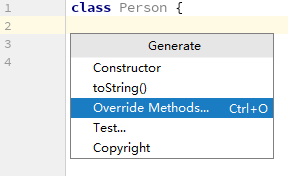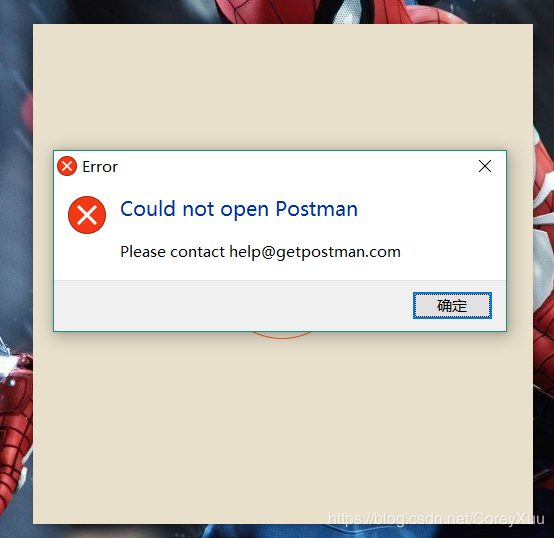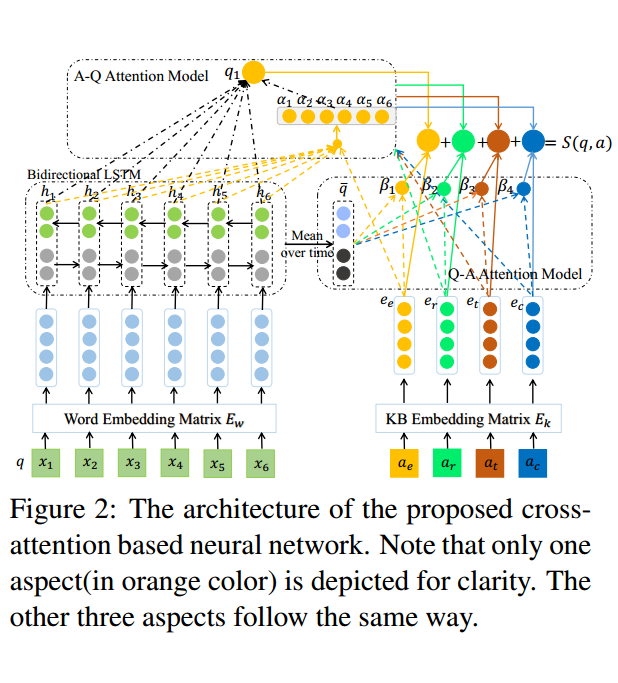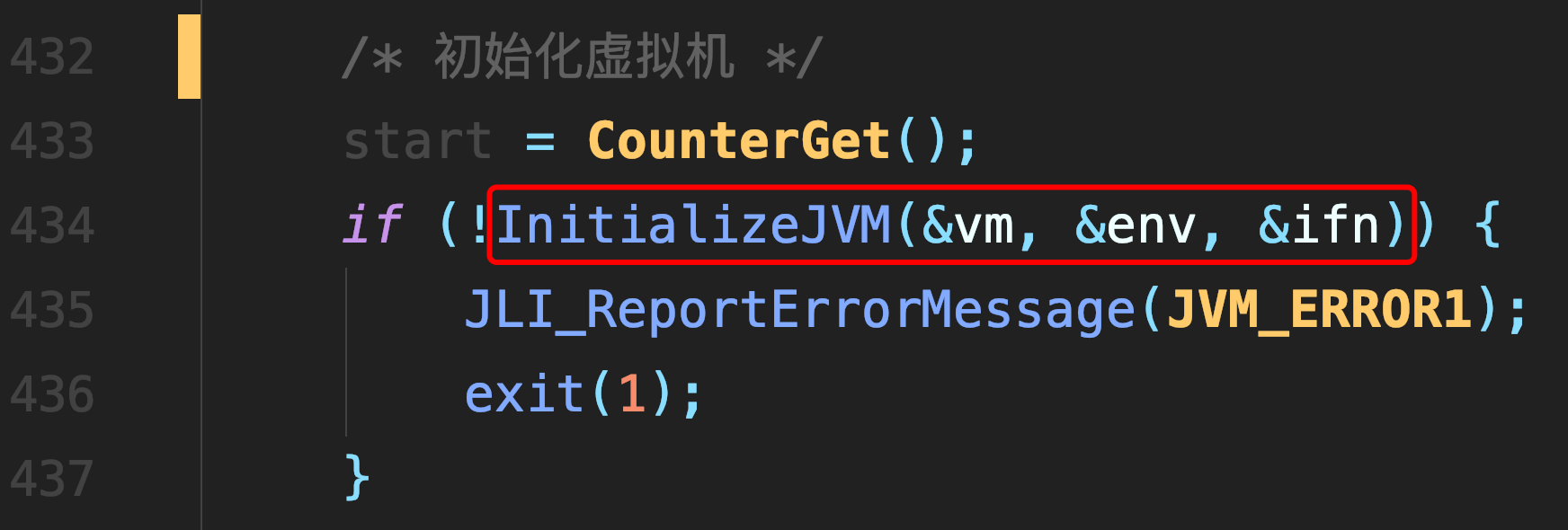# jdk1.7 HashMap中的致命错误：循环链表

## jdk1.7 HashMap中的"致命错误"：循环链表

### jdk1.7 HashMap结构图

#### jdk1.7是数组+链表的结构### jdk1.7版本中主要存在两个问题

1. 头插法会造成循环链表的情况

2. 链表过长，会导致查询效率下降

### jdk1.8版本针对jdk1.8进行优化

1. 使用尾插法，消除出现循环链表的情况
2. 链表过长后，转化为红黑树，提高查询效率

### 循环链表的产生

#### resize函数

void resize(int newCapacity)
{
Entry[] oldTable = table;
int oldCapacity = oldTable.length;
......
//创建一个新的Hash Table
Entry[] newTable = new Entry[newCapacity];
//将Old Hash Table上的数据迁移到New Hash Table上
transfer(newTable);
table = newTable;
}


#### transfer函数

transfer逻辑其实也简单，遍历旧数组，将旧数组元素通过头插法的方式，迁移到新数组的对应位置问题出就出在头插法

void transfer(Entry[] newTable)
{
//src旧数组
Entry[] src = table;
int newCapacity = newTable.length;

for (int j = 0; j < src.length; j++) {
Entry<K,V> e = src[j];
if (e != null) {
src[j] = null;
do {
Entry<K,V> next = e.next;
int i = indexFor(e.hash, newCapacity);
e.next = newTable[i];
newTable[i] = e;
e = next;
} while (e != null);//由于是链表，所以有个循环过程
}
}
}

static int indexFor(int h, int length){
return h&(length-1);
}


#### 下面举个实际例子

//下面详细解释需要用到这部分代码，所以先标号，将一下代码分为五个步骤
do {
1、Entry<K,V> next = e.next;
2、int i = indexFor(e.hash, newCapacity);
3、e.next = newTab[i];
4、newTable[i] = e;
5、e= next;
} while(e != null)

• #### 开始 $HashMap$容量设为2，加载阈值为 $2*0.75=1$• #### 线程 $T_2$和线程 $T_1$同时插入元素，由于阈值为1，所以都需要调用resize函数，进行扩容操作• #### 线程 $T_1$先阻塞于代码Entry<K,V> next = e.next;，之后线程 $T_2$执行完扩容操作• #### 之后线程 $T_1$被唤醒，继续执行，完成一次循环后

开始 $e\rightarrow3$, $next\rightarrow7$, 执行下面代码后， $e\rightarrow 7$, $next\rightarrow3$

2、int i = indexFor(e.hash, newCapacity);
3、e.next = newTab[i];
4、newTable[i] = e;
5、e= next;
1、Entry<K,V> next = e.next;• #### 线程 $T_1$执行第二次循环后

开始 $e\rightarrow 7$, $next \rightarrow 3$, 执行以下代码后， $e\rightarrow3$, $next \rightarrow null$

2、int i = indexFor(e.hash, newCapacity);
3、e.next = newTab[i];
4、newTable[i] = e;
5、e= next;
1、Entry<K,V> next = e.next;• #### 线程T1执行第三次循环后，形成死循环

开始 $e\rightarrow 3$, $next \rightarrow null$, 执行以下代码后， $e\rightarrow null$

2、int i = indexFor(e.hash, newCapacity);
3、e.next = newTab[i];
4、newTable[i] = e;
5、e= next;
1、Entry<K,V> next = e.next;### 参考文献

JDK1.7 HashMap 导致循环链表

##### 热门推荐
•浏览(654)
•浏览(563)
•浏览(547)
•浏览(480)
•浏览(424)
•浏览(419)
•浏览(381)
•浏览(366)
•浏览(362)
•浏览(344)